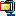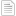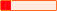#### Torrent DetailsNAME
Handbook of Mathematics for Engineers and Scientists.torrent
CATEGORY
eBooks
INFOHASH
889927af6b64e14f234dd9a6cb88205441bf4276
SIZE
10 MB in 4 files
Uploaded on 24-08-2018 by our crawler pet called "Spidey".
SWARM
0 seeders & 0 peers
RATING

#### Description

Description

The Handbook of Mathematics for Engineers and Scientists covers the main fields of mathematics and focuses on the methods used for obtaining solutions of various classes of mathematical equations that underlie the mathematical modeling of numerous phenomena and processes in science and technology. To accommodate different mathematical backgrounds, the preeminent authors outline the material in a simplified, schematic manner, avoiding special terminology wherever possible.

Organized in ascending order of complexity, the material is divided into two parts. The first part is a coherent survey of the most important definitions, formulas, equations, methods, and theorems. It covers arithmetic, elementary and analytic geometry, algebra, differential and integral calculus, special functions, calculus of variations, and probability theory. Numerous specific examples clarify the methods for solving problems and equations. The second part provides many in-depth mathematical tables, including those of exact solutions of various types of equations.

This concise, comprehensive compendium of mathematical definitions, formulas, and theorems provides the foundation for exploring scientific and technological phenomena.

#### Files in this torrent

FILENAMESIZEHandbook of Mathematics for Engineers and Scientists.zip10.5 MBTorrent_downloaded_from_Demonoid_-_www.demonoid.pw_.txt59 B[Bookflare.net] - Visit for more books.txt29 B[TGx]Downloaded from torrentgalaxy.org .txt524 B

#### Alternative Torrents for 'Handbook of Mathematics for Engineers and Scientists'.

TORRENT NAME ADDED SIZE SEEDS PEERS HEALTH8/24/2018 10 MB 0 02/12/2018 8 MB 0 011/10/2017 8 MB 0 0